# MCQs on Electric Circuits

##### Page 8 of 63. Go to page 1 2 3 4 5 6 7 8 9 10 11 12 13 14 15 16 17 18 19 20 21 22 23 24 25 26 27 28 29 30 31 32 33 34 35 36 37 38 39 40 41 42 43 44 45 46 47 48 49 50 51 52 53 54 55 56 57 58 59 60 61 62 63
01․ In parallel RLC circuit under resonance condition the value of current is
Maximum
Minimum
Zero
None of the above

In RLC parallel circuit under resonance condition the value of inductive susceptance is equal to capacitive susceptance and they will cancel each other. Therefore the value of admittance is minimum and impedance is maximum. Hence current is minimum in this condition.

02․ For ideal tank circuit, what is the value of dynamic admittance?
0
Both
None of the above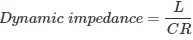For ideal tank circuit R value is zero. Therefore dynamic impedance is infinite and dynamic admittance is zero.

03․ For ideal tank circuit what is the value of dynamic impedance?
0
Both
None of the aboveFor ideal tank circuit R value is zero. Therefore dynamic impedance is infinite and dynamic admittance is zero.

04․ In series RLC circuit to get Q>1, which of the following is required?
XL > R
XL < R
XL = R
All of the above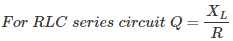So, to get Q > 1, XL > R.

05․ In RLC parallel circuit to get Q>1 which of the following is required?
XL>R
XL<R
XL=R
All of the above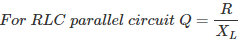In this case to get Q >1, R must be greater than XL.

06․ By which of the following elements transients will not occur?
R
L
C
All of the above

Resistance will not store the energy and it will allow sudden changes of voltage or current. Therefore transients will not occur in resistance. Transients will occur in inductance and capacitance only.

07․ Which of the following elements is/are called dynamic element/s?
R.
L.
C.
Both L and C.

Dynamic elements are nothing but energy storage elements, which will show transient response when they are connected to DC or AC.

08․ For steady state current inductor acts as
Short circuit
Open circuit
Voltage source
Current source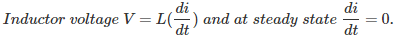Therefore at steady state current, inductor voltage is zero. So it will acts as short circuit for steady state current.

09․ In RL series circuit R=2Ω and L=10mH and applied voltage is 10V DC. Then find the current in the network?
0A
10A
5A
1A

For DC voltage inductor acts as a short circuit at steady state.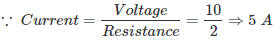10․ In steady state condition capacitor acts as a
short circuit
open circuit
voltage source
current source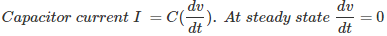Hence at steady state voltage there is no current. So, for steady state voltage capacitor acts as an open circuit.

<<<678910>>>